### Home > CALC > Chapter 5 > Lesson 5.2.4 > Problem5-96

5-96.
1. Simplify the following without a calculator. Homework Help ✎1.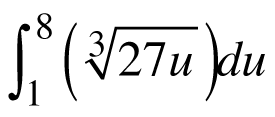2.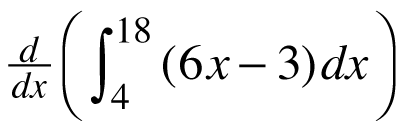3.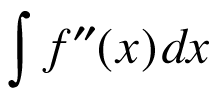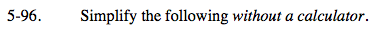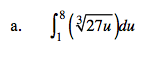Before integrating, rewrite the integrand with a fractional exponent. Note that both the 27 and the u are beneath the radical sign!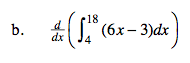The Fundamental Theorem of Calculus, part 1 states that the derivative of an indefinite integral gives you the original function. But this is the derivative of a DEFINITE integral.

0
Explain.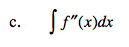$=\int \frac{d}{dx}f'(x)dx=\underline{ \ \ \ \ \ \ \ \ \ \ \ \ } +C$

Think: Fundamental Theorem of Calculus, part 1.